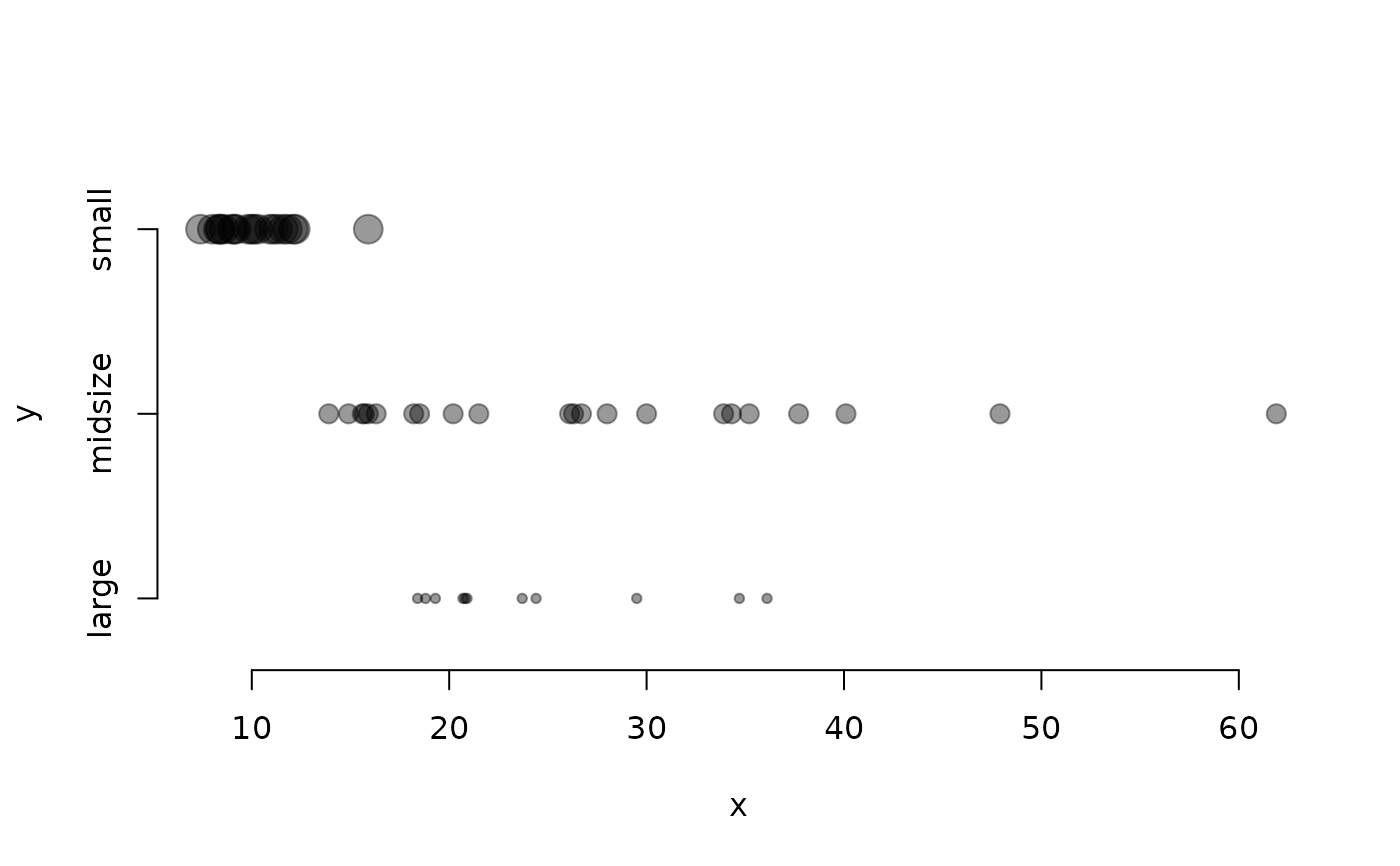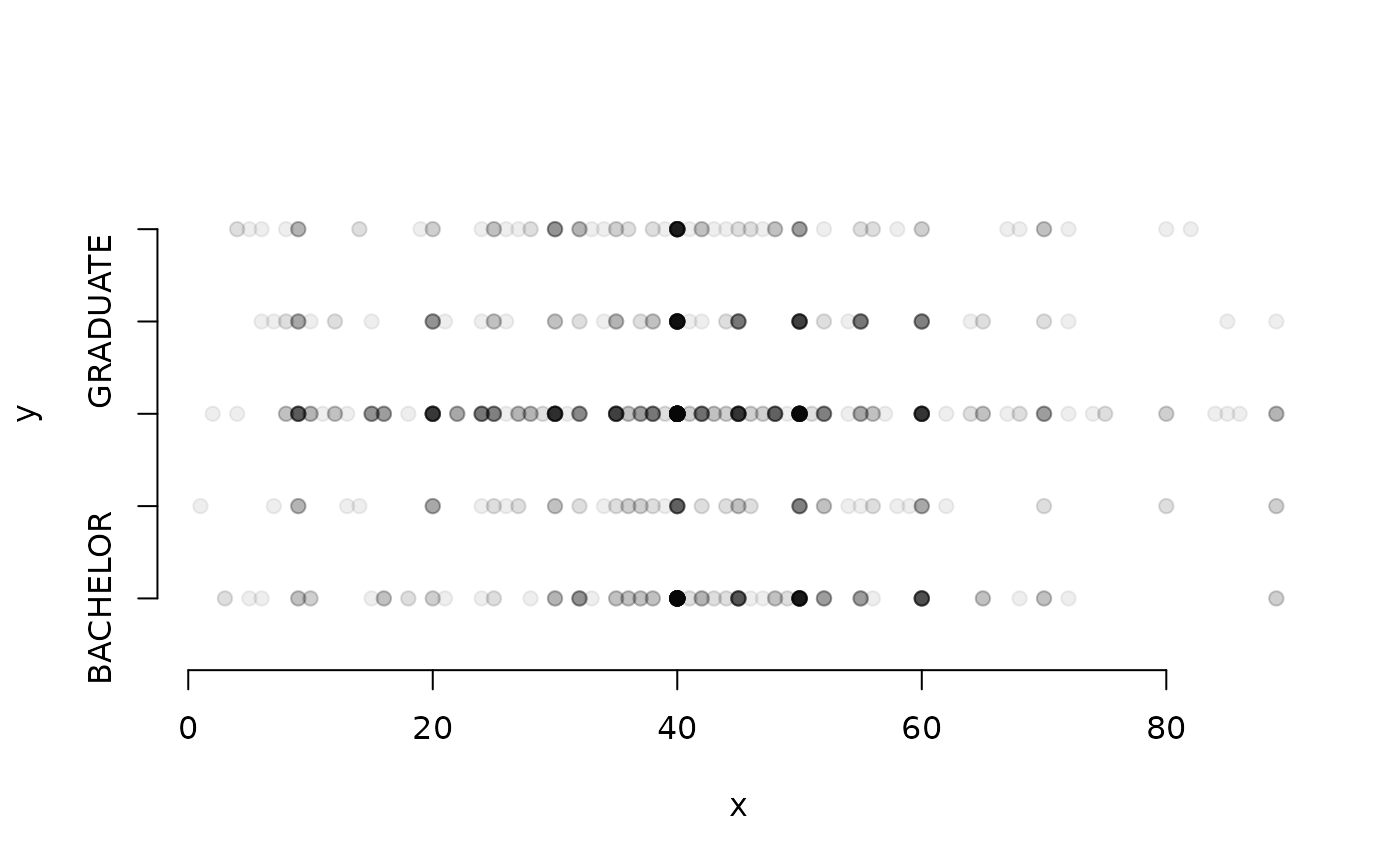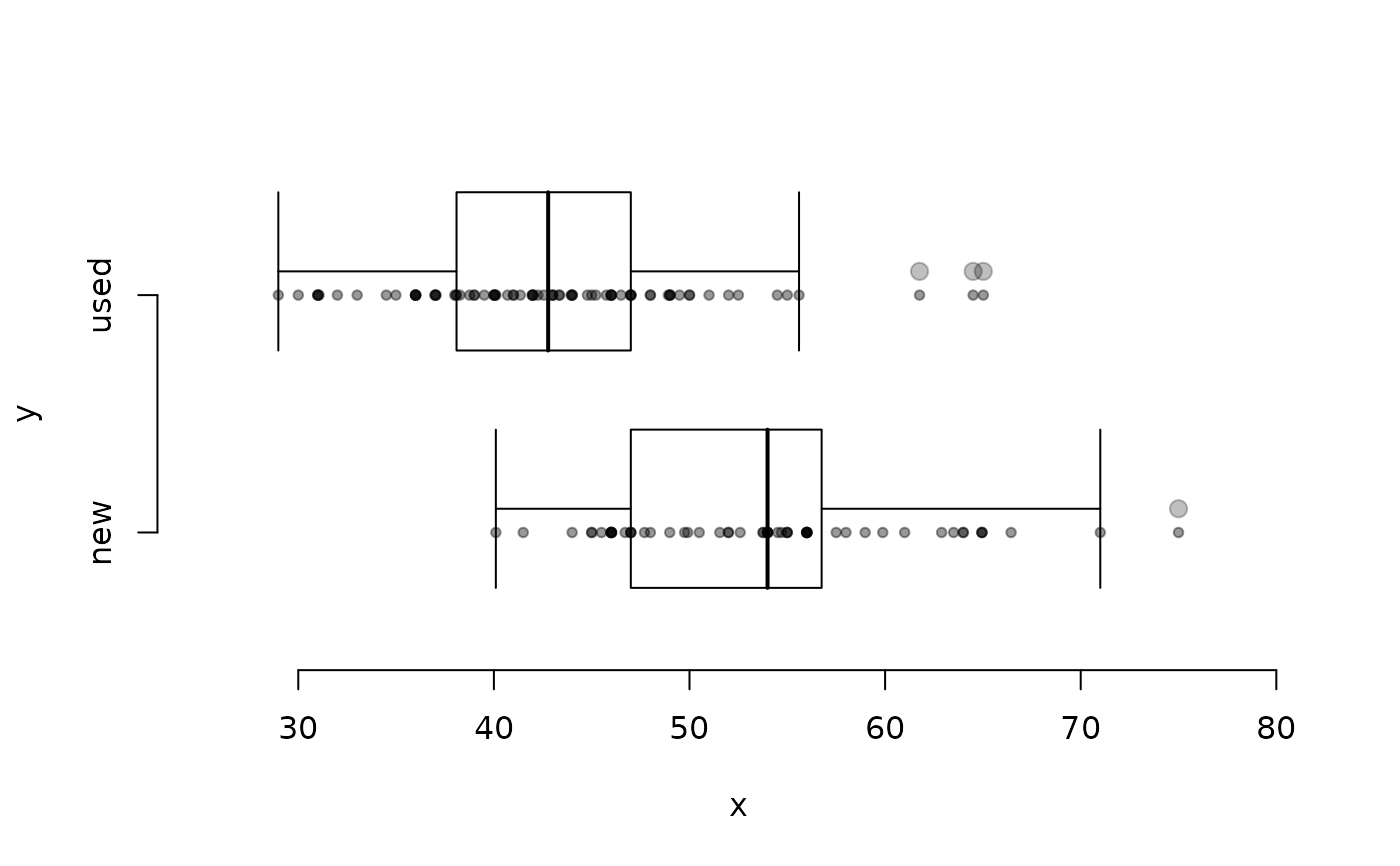Plot observations as dots.

## Usage

dotPlot(
x,
fact = NULL,
vertical = FALSE,
at = 1,
key = NULL,
pch = 20,
cex = 1.5,
axes = TRUE,
xlim = NULL,
ylim = NULL,
...
)

## Arguments

x

A numerical vector.

fact

A character or factor vector defining the grouping for data in x.

vertical

If TRUE, the plot will be oriented vertically.

at

The vertical coordinate of the points, or the horizontal coordinate if vertical=TRUE. If fact is provided, then locations can be specified for each group.

key

The factor levels corresponding to at, pch, col, and cex.

pch

Plotting character. If fact is given, then different plotting characters can be specified for each factor level. If key is specified, the elements of pch will correspond to the elements of key.

col

Plotting character color. If fact is given, then different colors can be specified for each factor level. If key is specified, the elements of col will correspond to the elements of key.

cex

Plotting character size. If fact is given, then different character sizes can be specified for each factor level. If key is specified, the elements of cex will correspond to the elements of key.

If TRUE, then the points are added to the plot.

axes

If FALSE, no axes are plotted.

xlim

Limits for the x axis.

ylim

Limits for the y axis.

...

Additional arguments to be passed to plot if add=FALSE or points if add=TRUE.

histPlot, densityPlot, boxPlot

David Diez

## Examples


library(dplyr)

# Price by type
dotPlot(cars93$price, cars93$type,
key = c("large", "midsize", "small"),
cex = 1:3
)# Hours worked by educational attainment or degree
gss2010_nona <- gss2010 %>%
filter(!is.na(hrs1) & !is.na(degree))

dotPlot(gss2010_nona$hrs1, gss2010_nona$degree,
)# levels reordered
dotPlot(gss2010_nona$hrs1, gss2010_nona$degree,
key = c("LT HIGH SCHOOL", "HIGH SCHOOL", "BACHELOR", "JUNIOR COLLEGE", "GRADUATE")
)# with boxPlot() overlaid
dotPlot(mariokart$total_pr, mariokart$cond,
ylim = c(0.5, 2.5), xlim = c(25, 80), cex = 1
)
boxPlot(mariokart$total_pr, mariokart$cond,